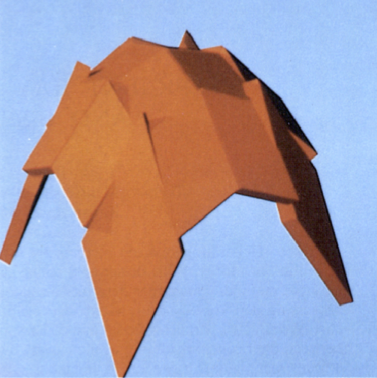# Efficient Ray Tracing for Bezier and B-Spline Surfaces

#### Abstract:

Generating realistic pictures by raytracing requires intersecting the objects with many rays (1~ million or more). With Bezier or B-Spline surfaces as objects the intersections must be calculated by an iterative method. This paper describes an algorithm which performs these calculations efficiently. In a preprocessing step the surface is subdivided adaptively into parts and a tight enclosure is calculated for each part. We selected parallelepipeds (first order approximations) as enclosures, their orientation and the angles between their edges are chosen in such a way that they enclose the respective part as tightly as possible, they are not rectangular in general. A binary tree built with these enclosures allows to test very fast which parts of the surface may be hit by a given ray. The leaves of the tree contain small, almost plane parts of the surface. For each part a linear approximation is calculated, this is a parallelogram, in general not rectangular. For each ray which hits the enclosure the intersection with this approximation is calculated first, yielding an accurate starting point for the following iteration.

Date of publication: Jul - 1993